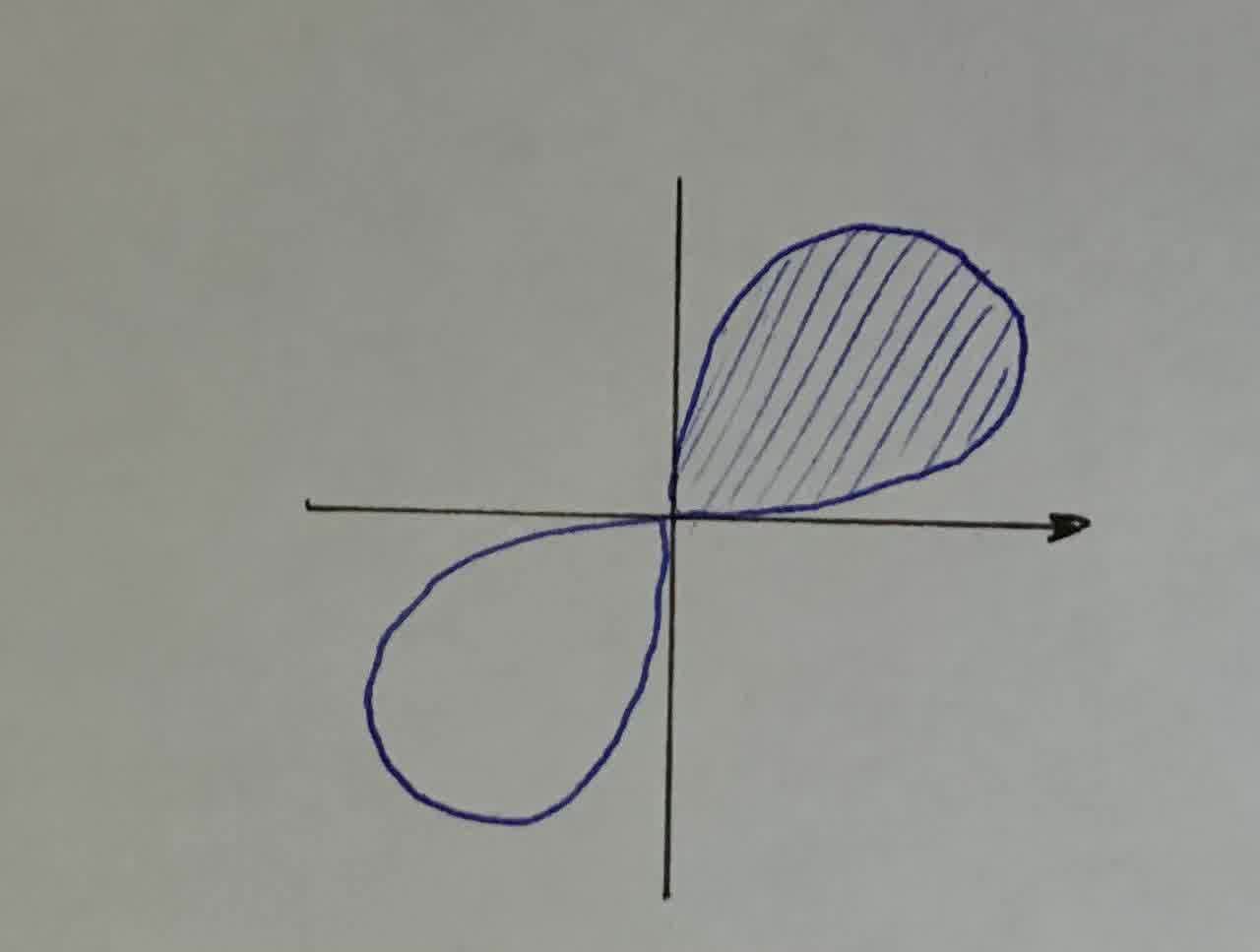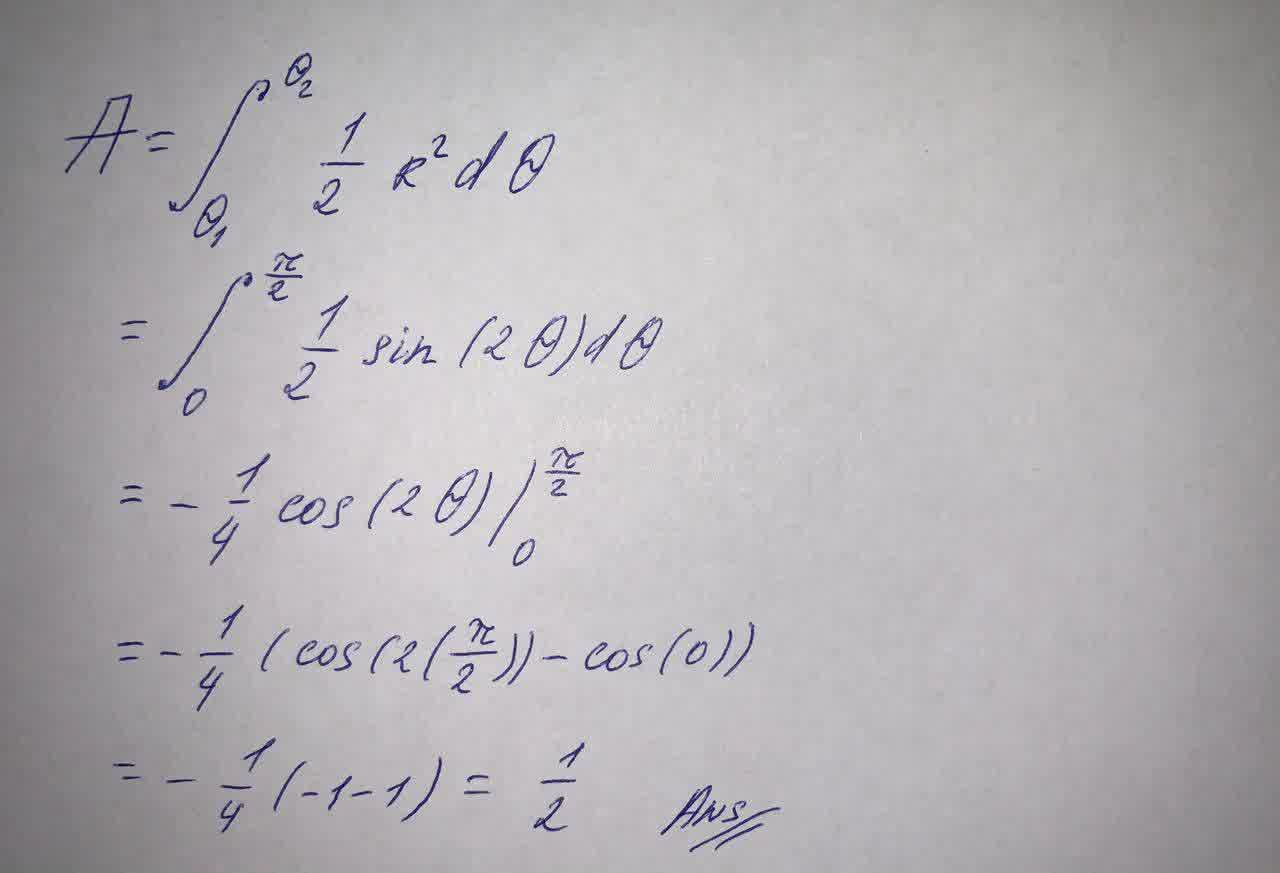Question# Find the area of the shaded region. r^2 = \sin (2 \theta)

Analytic geometry
ANSWEREDFind the area of the shaded region. $$r^2 = \sin (2 \theta)$$